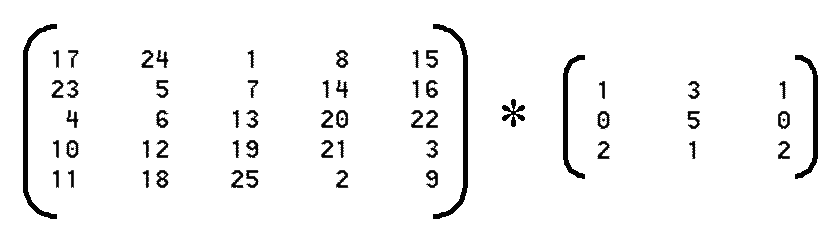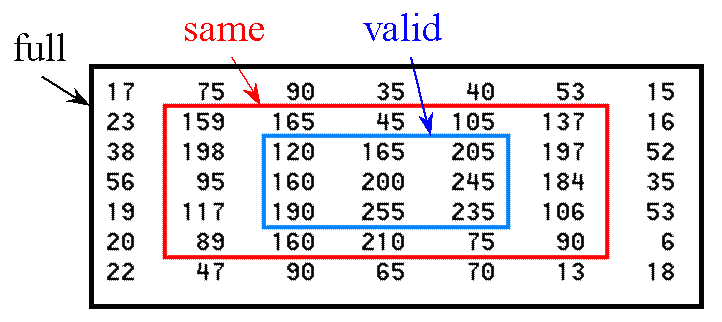# 2D Convolutionconv2

Perform two-dimensional convolution

Syntax

```C = conv2(A,B)
C = conv2(hcol,hrow,A)
C = conv2(...,`shape`)
```

Description

`C = conv2(A,B)` performs the two-dimensional convolution of matrices `A` and `B`, returning the result in the output matrix `C`. The size in each dimension of `C` is equal to the sum of the corresponding dimensions of the input matrices minus one. That is, if the size of `A` is `[ma,mb]` and the size of `B` is `[mb,nb]`, then the size of `C` is `[ma+mb-1,na+nb-1]`.

`C = conv2(hcol,hrow,A)` convolves `A` separably with `hcol` in the column
direction and `hrow` in the row direction. `hcol` and `hrow` are both vectors.

`C = conv2(...,``shape``)` returns a subsection of the two-dimensional convolution, as specified by the `shape` parameter. `shape` is a string with one of these values:

• `'full'` (the default) returns the full two-dimensional convolution.
• `'same'` returns the central part of the convolution of the same size as `A`.
• `'valid'` returns only those parts of the convolution that are computed without the zero-padded edges. Using this option, `size(C) = [ma-mb+1,na-nb+1]` when `size(A)` > `size(B)`.

For image filtering, `A` should be the image matrix and `B` should be the filter (convolution kernel) if the `shape` parameter is '`same'` or '`valid'`. If the `shape` parameter is '`full'`, the order does not matter, because full convolution is commutative.

Class Support

All vector and matrix inputs to `conv2` can be of class `double` or of any integer class. The output matrix `C` is of class `double`.

Remarks

`conv2` is a function in MATLAB.

## Example

```>> A=magic(5)

A =

17    24     1     8    15
23     5     7    14    16
4     6    13    20    22
10    12    19    21     3
11    18    25     2     9

>> B=[1 3 1; 0 5 0; 2 1 2]

B =

1     3     1
0     5     0
2     1     2

>> C1 = conv2(A,B,'full')

C1 =

17    75    90    35    40    53    15
23   159   165    45   105   137    16
38   198   120   165   205   197    52
56    95   160   200   245   184    35
19   117   190   255   235   106    53
20    89   160   210    75    90     6
22    47    90    65    70    13    18

>> C2 = conv2(A,B,'same')

C2 =

159   165    45   105   137
198   120   165   205   197
95   160   200   245   184
117   190   255   235   106
89   160   210    75    90

>> C3 = conv2(A,B,'valid')

C3 =

120   165   205
160   200   245
190   255   235

```

## What to do with the borders?

Since convolution is a neigborhood operation, the question arises of how to handle pixels that have no neighbors, namely those near the edge or borders of the image. There is no absolutely right way to handle this problem. Following are some suggestions based on common practices.

### (1) Valid region

Make the image big enough so that there is a guard ring around the region of interest. That is, use only the valid portion of the convolution. The resulting image will be smaller by the width of the convolution operator, as suggested by the outlined region below:### (2) Zero surround

Assume the image is embedded in a sea of zeros, as shown below. This is the assumption made by the full and same methods of `conv2`.### (3) Nearest neighbor

Assume that the pixels just outside the image are the same as their nearest neighbor inside the image. This is the situation portrayed below (obtained with `add_border(rgb,'dup',width)`)### (4) Circular or cyclic convolution

Consider the extended image to be a tiled version of the original, and then convolve the central image using portions of the adjacent tiles at the borders.

The following matlab code constructs a tiled image:

```>> out = repmat(srgb,3,3);
```A cyclic border can also be constructed from `add_border(rgb,'circ',width)`.### (5) Fancy Extrapolation

One could contemplate using a fancy extrapolation scheme, perhaps based on cubic splines, to obtain pixels outside the original image. This really has no more justifcation than the other methods described above, and is no substitute for making the original image much larger than the region of interest.

```function out = add_border(inp,type,width)
%
%  add border of given type and width to
%  an image

if (nargin<3)
width = 10;
end

if (nargin<2)
type = 'zero';
end

s = size(inp);

switch type(1)
case 'z',
s(2)=width;
z = zeros(s);
tmp = [z, inp, z];
s = size(tmp);
s(1)=width;
z = zeros(s);
out = [z;tmp;z];
case 'd',
c = inp(:,1,:);
left = repmat(c,1,width);
c = inp(:,s(2),:);
right = repmat(c,1,width);
tmp = [left, inp, right];
s = size(tmp);
r = tmp(1,:,:);
top = repmat(r,width,1);
r = tmp(s(1),:,:);
bot = repmat(r,width,1);
out = [top;tmp;bot];
case 'c',
left = inp(:,1:width,:);
right = inp(:,(s(2)-width+1):s(2),:);
tmp = [right,inp,left];
s = size(tmp);
top = tmp(1:width,:,:);
bot = tmp((s(1)-width+1):s(1),:,:);
out = [bot;tmp;top];
otherwise,
error('Invalid input arguments.');
end
```

Maintained by John Loomis, last updated 14 Feb 2001